## Lucky Streak

I have some friends who like to gamble at the casinos. They told me their “winning” strategy is based on the concept of runs or streaks. Their idea is to recognize when they are in the middle of a streak and increase the amount bet. Their basic strategy (a bit generalized) is to bet a small amount until they get two “wins” in a row, and then increase the bet. And continue increasing the bet for every additional “win”.

Even if you don’t know probability, you can can very easily test this strategy in R.

## Simulating Wins

Here we will build a simple simulation that will randomly draw a series of “wins” and “losses” to mimic the casino. Say you are playing roulette or blackjack and have constant probability of winning. This also assumes the outcomes are independent, so past outcomes have no influence on current or future outcomes.

The first thing to do is the set-up. Here we set \(p\), the probability of winning on any hand and \(n\), the number of times played. Also, we set the seed for the random number generator so we get the same outcome every time we run the simulation (useful for classroom demos). You can follow along by copying and pasting into a script.

``````#-- Settings
set.seed(2016)               # set seed for RNG to allow replication
p = 0.50                     # probability of win
n = 1000                     # number of times played``````

Next, we use the function `sample()` to generate the random outcomes.

``````#-- Random sample of outcomes
x = sample(x = c('win', 'lose'), size=n, replace=TRUE, prob=c(p, 1-p))

#-- show/print the first few values in X
``##  "lose" "lose" "win"  "lose" "lose" "lose"``
``````#-- Get counts of outcomes
table(x)``````
``````## x
## lose  win
##  480  520``````

In this simulation, we got 520 wins and 480 losses.

## Run Lengths

To get the information on winning streaks, we can use the function `rle()`. To see the help page of an R function, put a `?` before the function name, i.e., `?rle`.

``````r = rle(x)       # ?rle returns a list of two elements: lengths and values
r                # show the results``````
``````## Run Length Encoding
##   lengths: int [1:472] 2 1 3 2 5 1 2 1 1 1 ...
##   values : chr [1:472] "lose" "win" "lose" "win" "lose" "win" ...``````

This gives us the run lengths (or length of winning and losing streaks).

We can use the `table()` function again to get a summary of the streaks

``table(streak=r\$lengths, r\$values)``
``````##
## streak lose win
##     1   111 108
##     2    64  54
##     3    29  31
##     4    19  20
##     5     7  13
##     6     2   8
##     7     3   0
##     9     0   2
##     10    1   0``````

This shows the longest streak was 10 losses in a row.

## Evaluating the strategy

With a little more effort we can evaluate how well the gambler's strategy would have done. First, we will make a function that will calculate the amount bet at each stage. The betting strategy is: if we get two “wins” in a row, then increase the bet. And continue increasing for every additional “win”. At the next “lose”, reset the bet back to initial value. Here, I will set the initial bet at `1` unit and the increase at `.5` units.

``````make_bets <- function(x, initial=1, increase=1/2){

#- initialize
n = length(x)               # length of x vector
bet = numeric(n)            # create numeric vector of length n
bet = initial            # set initial bet value
streak = 0                  # set winning streak at 0

#- loop
for(i in 2:n){
if(x[i-1] == 'win'){
streak = streak + 1
bet[i] = ifelse(streak >= 2, bet[i-1] + increase, bet[i-1])
}
if(x[i-1] == 'lose'){
streak = 0
bet[i] = initial
}
}
return(bet)
}``````

Running the betting function gives us the bets we would have made (if we stuck with our strategy)

``````bet = make_bets(x)                      # get the bets

head(data.frame(x,bet), 10)             # look at first 10 value``````
``````##       x bet
## 1  lose 1.0
## 2  lose 1.0
## 3   win 1.0
## 4  lose 1.0
## 5  lose 1.0
## 6  lose 1.0
## 7   win 1.0
## 8   win 1.0
## 9  lose 1.5
## 10 lose 1.0``````
``mean(bet)                               # average bet made``
``##  1.2945``
``````plot(bet, typ='l')                      # plot results
abline(h=mean(bet), col='red', las=1)   # add red horizontal line``````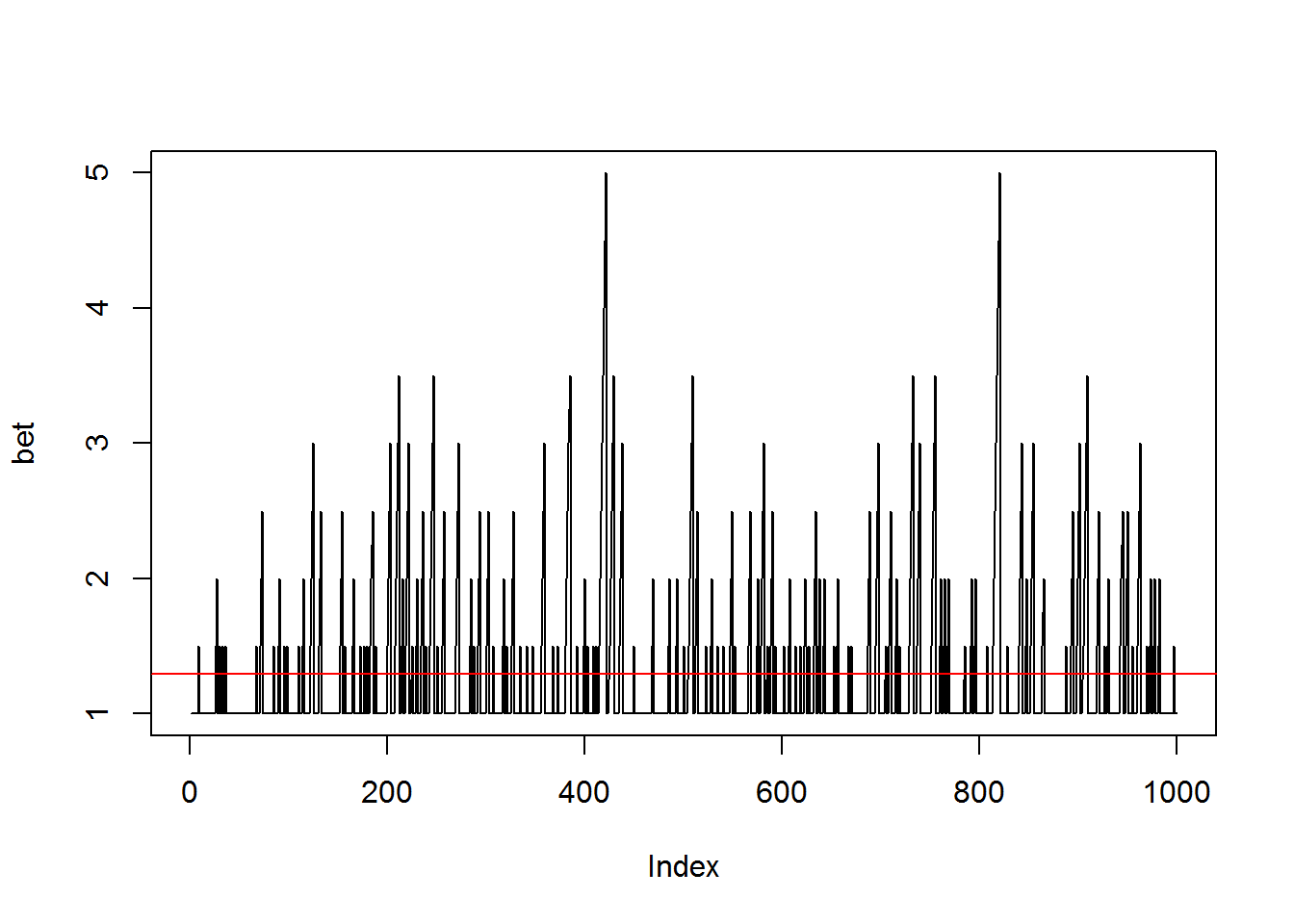### Results

We can see how many times we would have won or lost with each bet amount

``table(bet=bet, outcome=x)``
``````##      outcome
## bet   lose win
##   1    352 364
##   1.5   54  74
##   2     31  43
##   2.5   20  23
##   3     13  10
##   3.5    8   2
##   4      0   2
##   4.5    0   2
##   5      2   0``````

To see how much money we would have made (or lost), we can just apply the profit for each game. If we lose, the profit is `-bet`, but if we win the profit is `payoff*bet`.

``````payoff = 0.95   # for every 1 unit bet, we get 0.95 units of profit

profit = ifelse(x=='win', bet*payoff, -bet)``````

Put it all together in a data frame

``````y = data.frame(x, bet, profit)
``````##       x bet profit
## 1  lose 1.0  -1.00
## 2  lose 1.0  -1.00
## 3   win 1.0   0.95
## 4  lose 1.0  -1.00
## 5  lose 1.0  -1.00
## 6  lose 1.0  -1.00
## 7   win 1.0   0.95
## 8   win 1.0   0.95
## 9  lose 1.5  -1.50
## 10 lose 1.0  -1.00
## 11 lose 1.0  -1.00
## 12 lose 1.0  -1.00
## 13 lose 1.0  -1.00
## 14  win 1.0   0.95
## 15 lose 1.0  -1.00
## 16 lose 1.0  -1.00
## 17  win 1.0   0.95
## 18 lose 1.0  -1.00
## 19  win 1.0   0.95
## 20 lose 1.0  -1.00``````

Now we can visualize the outcome

``plot(profit, type='h')     # profit for each outcome``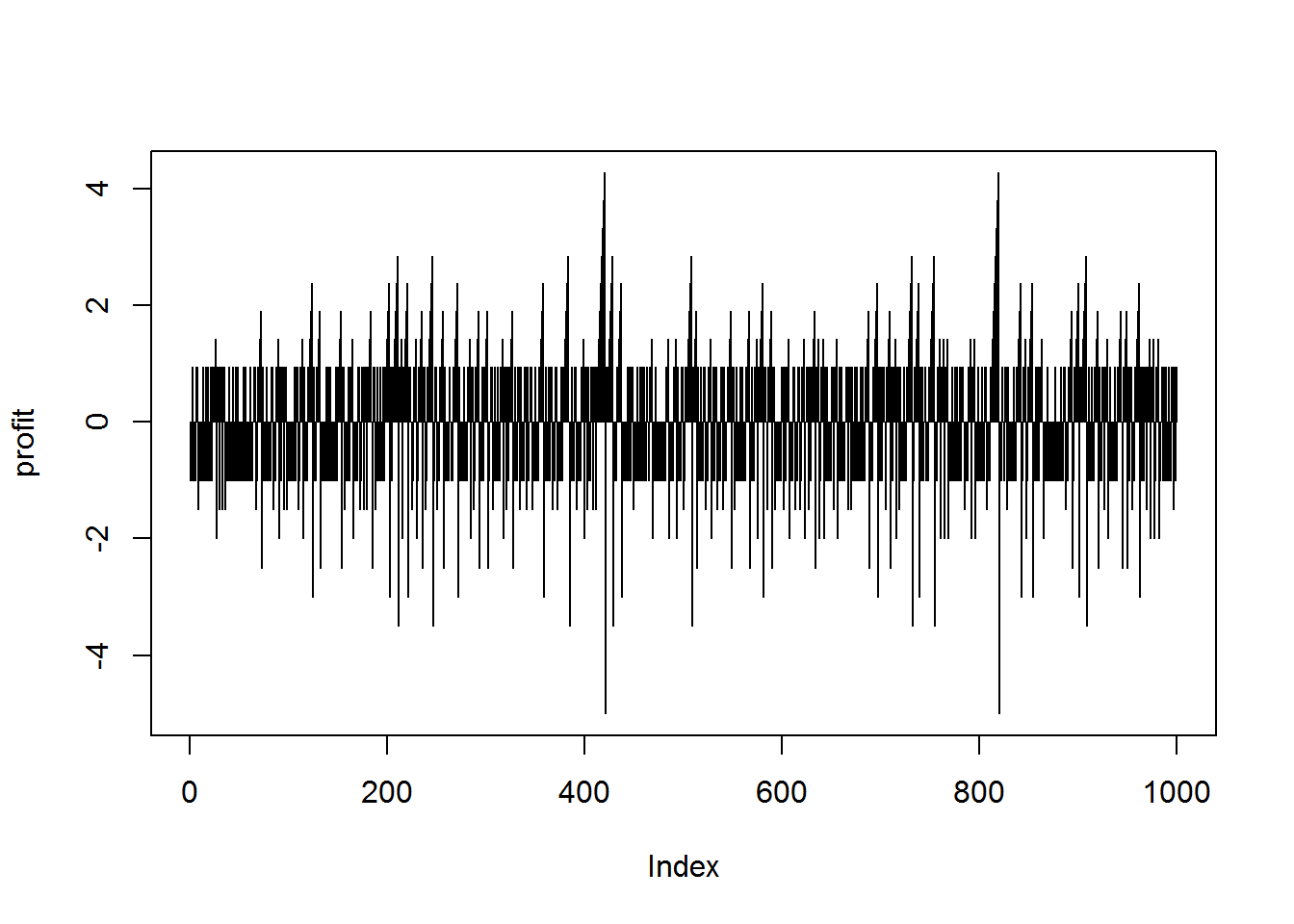``````plot(cumsum(profit),       # cumulative sum
type='l',             # set plot type to line
las=1,                # put y axis labels horizontal
xlab="number of games", # change x label
ylab='Total Profit')  # change y label

abline(h=0, col="lightgray")``````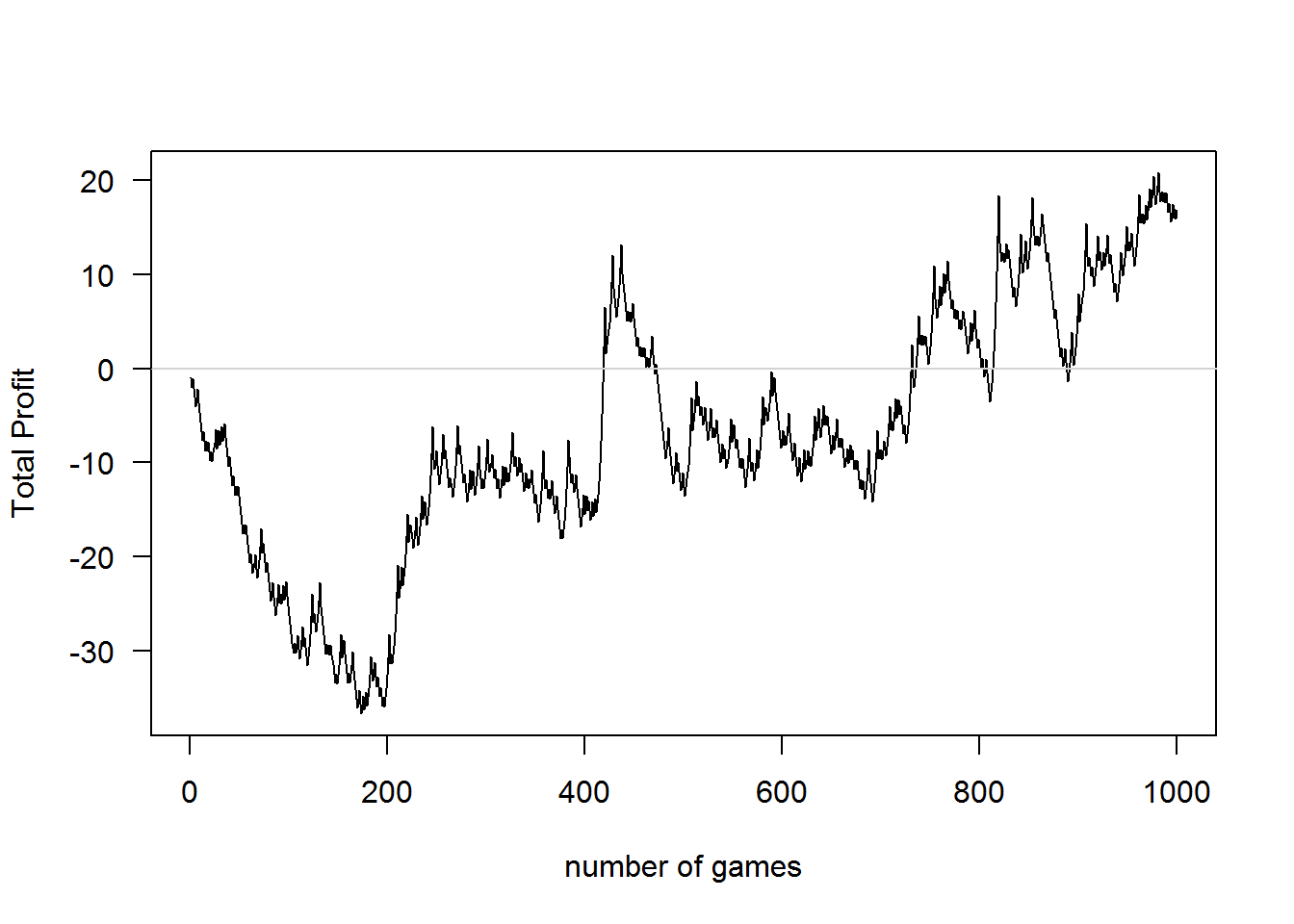Congratulations, we would have come out a winner! Well, if we would have kept playing 1000 times. The worst we would have done was stop at on the game 174 giving us a total profit of -36.68 units.

But what if we just bet 1 unit every time, without considering the streaks?

``profit_1 = ifelse(x=='win', 1*payoff, -1)``
``lines(cumsum(profit_1), col="blue") # add the new total profit to plot``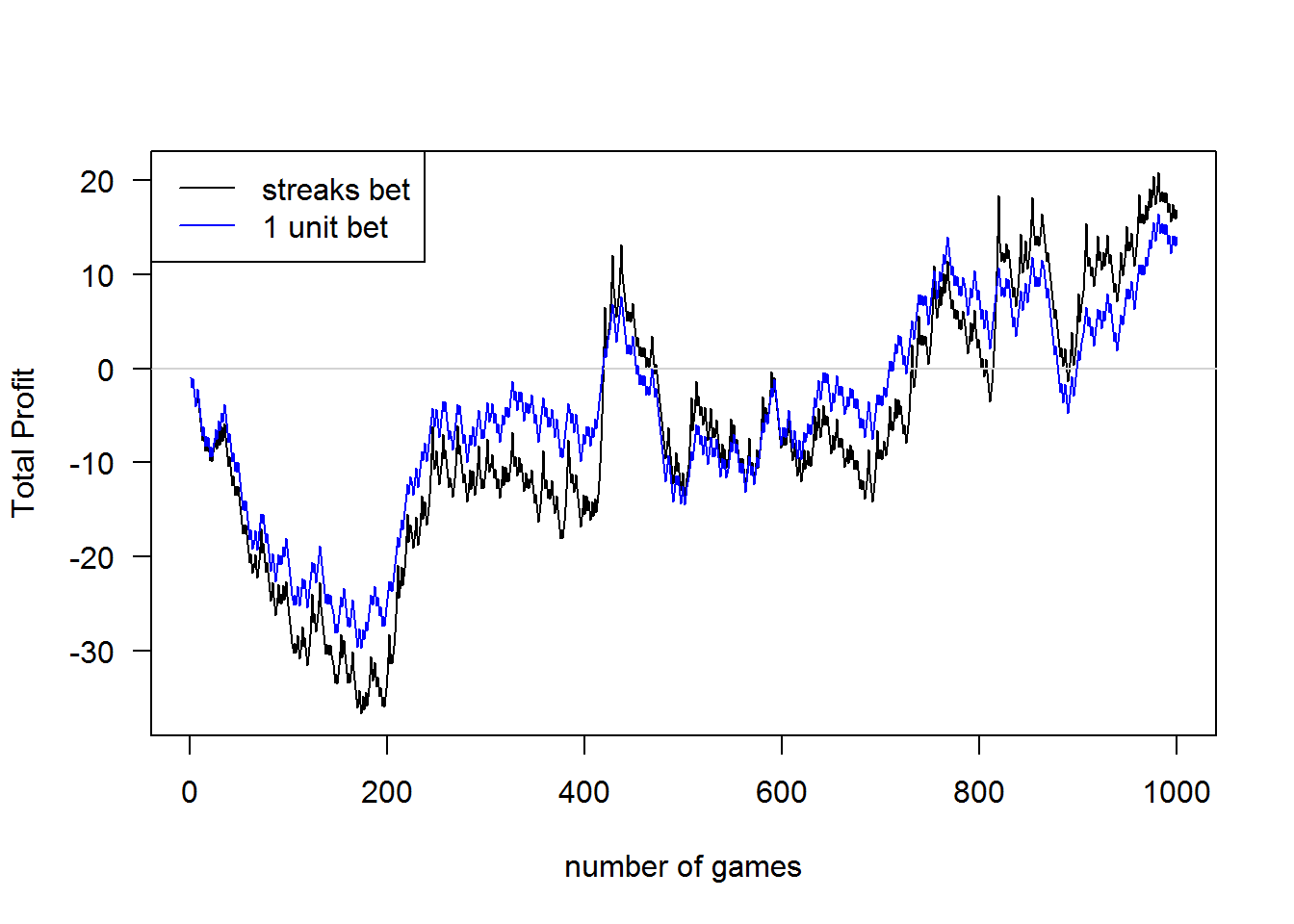Or better yet, we should have bet 2 units each time!

``profit_2 = ifelse(x=='win', 2*payoff, -2)``
``lines(cumsum(profit_2), col="orange")``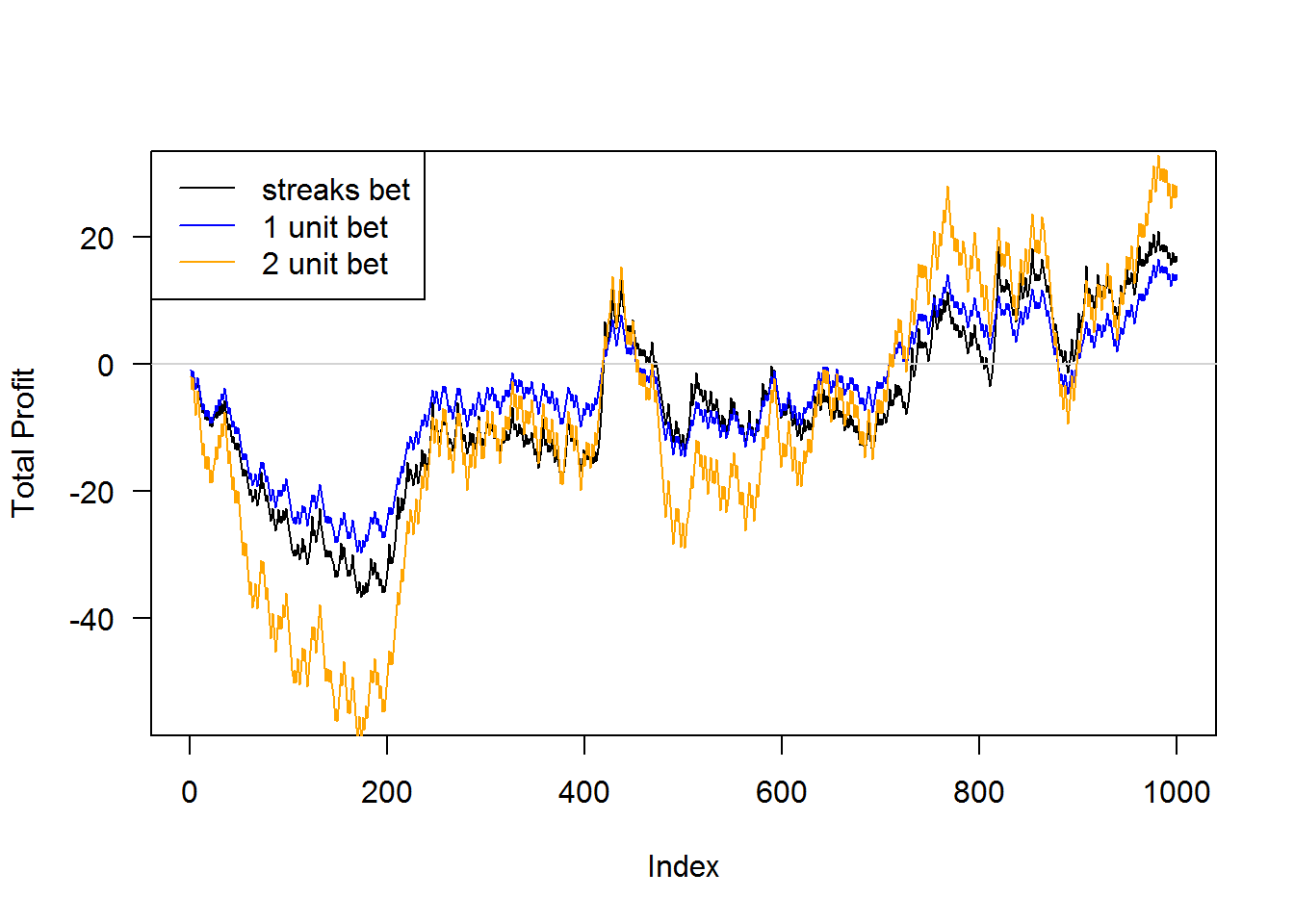Again, think about what would happen if we didn’t play 1000 games? Using the 2 unit bet, we would have a low of -59.4 units if we didn’t continue playing.

## Replicable Patterns

OK, so we won with our betting strategy. But we would have won with the naive betting strategy too. But, notice that we only would have come out ahead if we played over 700 games or so.

Are we seeing a real pattern? Or did this just happen to work out for us? Gamblers are notorious for only remembering the winning days, and forgetting the losing ones. Here is where simulation and probability can really help.

This lesson uses simulation to evaluate the strategy, but we could also use probability concepts to come to the same conclusion.

In short, this is not a wise strategy. Look up gambler's fallacy and reverse martingale strategy if you are interested in the details.

### Observation #1

If the strategy were to work, then having a series of wins should increase the probability of getting another win. We said in advance that these were independent outcomes, so by definition this shouldn’t be. If we flip a coin 5 times and get all heads, is the next flip due to be tails? Or are we on a streak and it is more likely to be heads? Or is it still 50-50 (for fair coin)?

We can test this. Here is a plot of the proportion of wins and losses given a certain event history.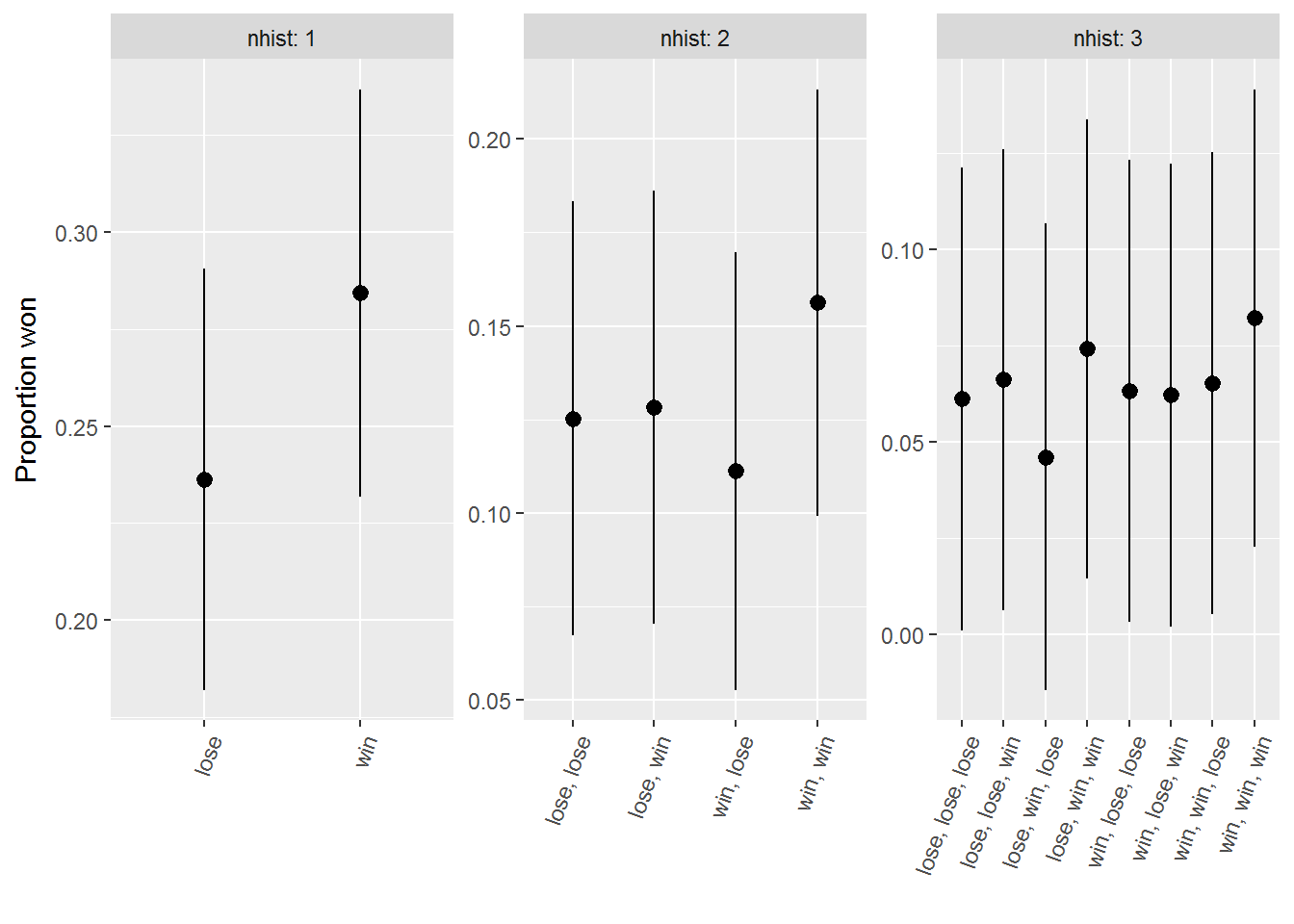This shows that there is no real pattern. The probability of getting a “win” is not dependent on the past results. The game, as expected, is memoryless. What happened in the past does not impact what will happen in the future.

### Observation #2

This simulates the game for one player who plays 1000 games. It turned out that if we played the full 1000 games, we would have come out ahead. But this is due to chance (or some call it luck), not a great strategy.

We can also simulate many gamblers. It looks like some of the gamblers would have come out with large profits, but some would have lost big too. You only hear from the ones that won big! This introduces a response bias whereby we can be lead to conclude a strategy is good because we only/mainly hear from the winners.

``````#-- Settings
set.seed(2016)       # set seed for RNG to allow replication
p = 0.50             # probability of win
n = 1000             # number of times played
payoff = 0.95        # for every 1 unit bet, we get 0.95 units of profit
ngamblers = 200      # number of gamblers

#-- simulation of all gamblers
Profit = Profit_1 = Profit_2 = matrix(NA, n, ngamblers)
for(i in 1:ngamblers){
x = sample(x = c('win', 'lose'), size=n, replace=TRUE, prob=c(p, 1-p))
bet = make_bets(x)                      # get the bets
Profit[,i] = ifelse(x=='win', bet*payoff, -bet)
Profit_1[,i] = ifelse(x=='win', 1*payoff, -1)
Profit_2[,i] = ifelse(x=='win', 2*payoff, -2)
}``````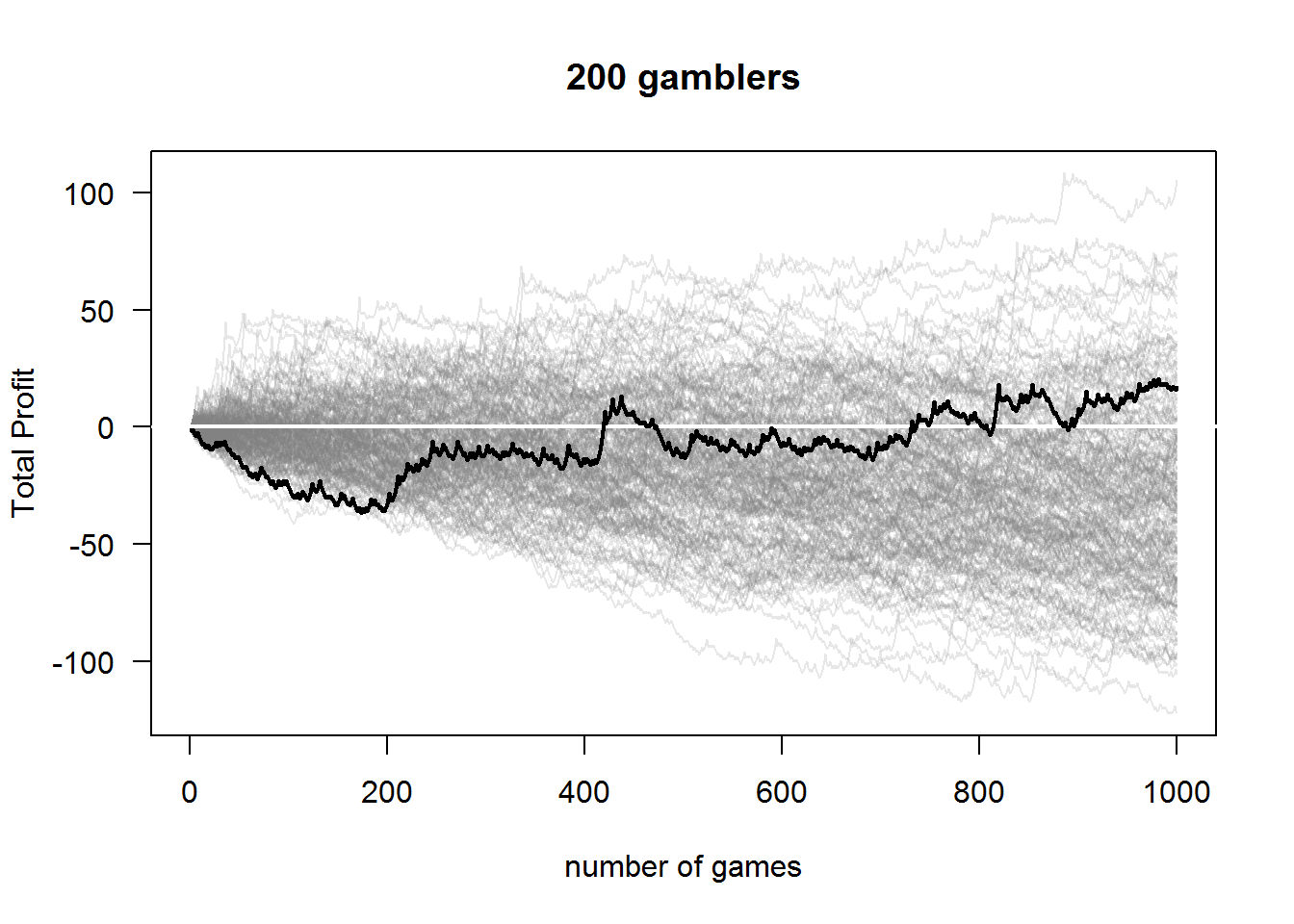The above plot shows the total profit functions for all 200 gamblers as well as our original outcome in bold. You should notice two important things. First, the variation grows as the number of games increase. The longer you play, the more variation in your profit. Second, and a bit more difficult to see from this plot, is that as the number of games increases more gamblers get negative profits.

To see this second property better, we can plot the average profit for our gamblers. The average profit is negative, so you can expect to be a loser.